# College Physics

Physics & Astronomy

## Quiz 12 :RelativityLooking for Introductory Physics Homework Help?

## Quiz 12 :Relativity

Showing 1 - 20 of 62A spacecraft with the shape of a sphere of diameter D moves past an observer on Earth with a speed of 0.5 c. What shape does the observer measure for the spacecraft as it moves past
Free
Essay

The length of the object is contracted along the direction of motion as the object is moving with relativistic speed is called the length contraction. The spacecraft with the shape of sphere is moving with speed 0.5c relative an observer on Earth. So the diameter of the spacecraft is reduced along the direction of spacecraft motion. Hence, the shape of the spacecraft is ellipse instead of spherical shape.If astronauts could travel at v = 0.950 c , we on Earth would say it takes (4.20/0.950) = 4.42 years to reach Alpha Centauri, 4.20 light-years away. The astronauts disagree. (a) How much time passes on the astronauts' clocks (b) What is the distance to Alpha Centauri as measured by the astronauts
Free
Essay

The expression for time interval measured in the moving frame of reference is,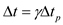Here,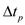is the time interval measured in the rest frame and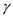is the relativistic factor.
The expression for the relativistic factor is,Here, v is the speed of the astronaut and c is the velocity of light.
(a)
Rearrange the equationforas follows:Substitutefor.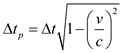The observer in the astronaut's frame measures the proper time and observer in the Earth's frame measures the dilated time.
Substitute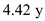for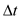and 0.950 c for v in the expression.Therefore, the proper time interval is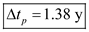.
(b)
The observer in the astronaut's frame measures the proper length and observer in the Earth's frame measures the contracted length.
The expression for the distance measured by the astronaut is,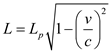Here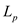is the proper length and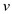is the speed of the astronaut.
Substitute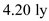forand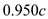for.Therefore, the distance to Alpha Centaury measured by the astronaut is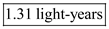.What two speed measurements will two observers in relative motion always agree upon
Free
Essay

Suppose the quasar moving with relativistic speed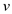and it is emitting a light. The speed of the light measured by the two observers is same even if they are moving relative to one another. Therefore, two observers always agree on the speed of light and the speed of one observer relative to the other because its magnitude is also same as measured by two observers.A meterstick moving at 0.900 c relative to the Earth's surface approaches an observer at rest with respect to the Earth's surface. (a) What is the meterstick's length as measured by the observer (b) Qualitatively, how would the answer to part (a) change if the observer started running toward the meterstick
EssayThe speed of light in water is 2.30 × 10 8 m/s. Suppose an electron is moving through water at 2.50 × 10 8 m/s. Does this particle speed violate the principle of relativity
EssayThe length of a moving spaceship is 28.0 m according to an astronaut on the spaceship. If the spaceship is contracted by 15.0 cm according to an Earth observer, what is the speed of the spaceship
EssayWith regard to reference frames, how does general relativity differ from special relativity
EssayAn astronaut at rest on Earth has a heart rate of 70 beats/min. When the astronaut is traveling in a spaceship at 0.90 c , what will this rate be as measured by (a) an observer also in the ship and (b) an observer at rest on Earth
EssayGive a physical argument that shows it is impossible to accelerate an object of mass m to the speed of light, even with a continuous force acting on it.
EssayThe average lifetime of a pi meson in its own frame of reference (i.e., the proper lifetime) is 2.6 × 10 8 s. If the meson moves with a speed of 0.98 c , what is (a) its mean lifetime as measured by an observer on Earth, and (b) the average distance it travels before decaying, as measured by an observer on Earth (c) What distance would it travel if time dilation did not occur
EssayIt is said that Einstein, in his teenage years, asked the question, "What would I see in a mirror if I carried it in my hands and ran at a speed near that of light " How would you answer this question
EssayAn astronaut is traveling in a space vehicle that has a speed of 0.500 c relative to Earth. The astronaut measures his pulse rate at 75.0 per minute. Signals generated by the astronaut's pulse are radioed to Earth when the vehicle is moving perpendicular to a line that connects the vehicle with an Earth observer. (a) What pulse rate does the Earth observer measure (b) What would be the pulse rate if the speed of the space vehicle were increased to 0.990 c
EssayList some ways our day-to-day lives would change if the speed of light were only 50 m/s.
EssayA muon formed high in Earth's atmosphere travels toward Earth at a speed v = 0.990 c for a distance of 4.60 km as measured by an observer at rest with respect to Earth. It then decays into an electron, a neutrino, and an antineutrino. (a) How long does the muon survive according to an observer at rest on Earth (b) Compute the gamma factor associated with the muon. (c) How much time passes according to an observer traveling with the muon (d) What distance does the muon travel according to an observer traveling with the muon (e) A third observer traveling toward the muon at c /2 measures the lifetime of the particle. According to this observer, is the muon's lifetime shorter or longer than the lifetime measured by the observer at rest with respect to Earth Explain.
EssayTwo identically constructed clocks are synchronized. One is put into orbit around Earth, and the other remains on Earth. (a) Which clock runs more slowly (b) When the moving clock returns to Earth, will the two clocks still be synchronized Discuss from the standpoints of both special and general relativity.
EssayA deep-space probe moves away from Earth with a speed of 0.80 c. An antenna on the probe requires 3.0 s, in probe time, to rotate through 1.0 rev. How much time is required for 1.0 rev according to an observer on Earth
EssayPhotons of light have zero mass. How is it possible that they have momentum
EssayThe proper length of one spaceship is three times that of another. The two spaceships are traveling in the same direction and, while both are passing overhead, an Earth observer measures the two spaceships to have the same length. If the slower spaceship has a speed of 0.350 c with respect to Earth, determine the speed of the faster spaceship.
EssayA car traveling at 35.0 m/s takes 26.0 minutes to travel a certain distance according to the driver's clock in the car. How long does the trip take according to an observer at rest on Earth Hint: The following approximation is helpful: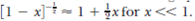.